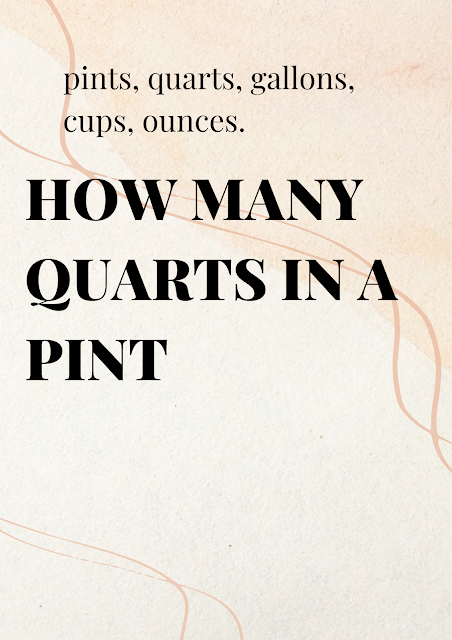# How many quarts in a pint? pints, quarts, gallons, cups, ounces.

In this article, we will cover the most common liquid measurements and their equivalents. By the end of this article, you will be able to confidently convert between pints, quarts, gallons, cups, and ounces.how many quarts in a pint

## How many quarts in a pint?

A pint is a unit of measurement for liquids that is equivalent to 16 fluid ounces. In terms of quarts, there are 2 pints in a quart. This means that a quart is equal to 32 fluid ounces. If you need to convert from pints to quarts, simply divide the number of pints by 2.

## How many cups in a pint?

In addition to pints, cups are another common unit of measurement for liquids. There are 2 cups in a pint, which means that a cup is equal to 8 fluid ounces. This is useful to know when you need to convert between cups and pints or vice versa.

## How many pints in a gallon?

A gallon is a larger unit of measurement for liquids that is equivalent to 128 fluid ounces. There are 8 pints in a gallon, which means that each pint is equal to 16 fluid ounces. This is useful to know when you need to convert between pints and gallons or vice versa.

## How many quarts in a gallon?

Similar to pints and gallons, quarts and gallons are also commonly used units of measurement for liquids. There are 4 quarts in a gallon, which means that each quart is equal to 32 fluid ounces. This is useful to know when you need to convert between quarts and gallons or vice versa.

## How many cups in a quart?

A quart is a smaller unit of measurement for liquids that is equivalent to 32 fluid ounces. There are 4 cups in a quart, which means that each cup is equal to 8 fluid ounces. This is useful to know when you need to convert between cups and quarts or vice versa:

• 1 quart = 4 cups
• 1 cup = 0.25 quarts

## How many ounces in a quart?

As mentioned earlier, a quart is equivalent to 32 fluid ounces. This means that if you need to convert from quarts to ounces, you simply need to multiply the number of quarts by 32:

• 1 quart = 32 ounces
• 1 ounce = 0.03125 quarts

## Pints abbreviation

When measuring liquids, it's common to use abbreviations to save time and space. The abbreviation for pint is "pt". This can be useful to know when reading recipes or measuring liquids in a kitchen.

## Pints to quarts to gallons

Now that we've covered the different liquid measurements and their equivalents, let's talk about how to convert between them. To convert pints to quarts, simply divide the number of pints by 2. To convert quarts to gallons, divide the number of quarts by 4. To convert pints to gallons, divide the number of pints by 8. Keep in mind that these are just basic conversion formulas and there are more complex conversions that may require additional steps:

• 1 gallon = 4 quarts
• 1 quart = 0.25 gallons

### Tablespoons to Quarts

If a recipe calls for tablespoons, but you need to convert it to quarts, you can use the following conversion:
• 1 quart = 64 tablespoons
• 1 tablespoon = 0.015625 quarts

In conclusion, understanding liquid measurements is an essential skill for anyone who enjoys cooking and baking. Knowing the equivalents between pints, quarts, gallons, cups, and ounces can make it easier to follow recipes and ensure that your final product turns out just right.

No Comment